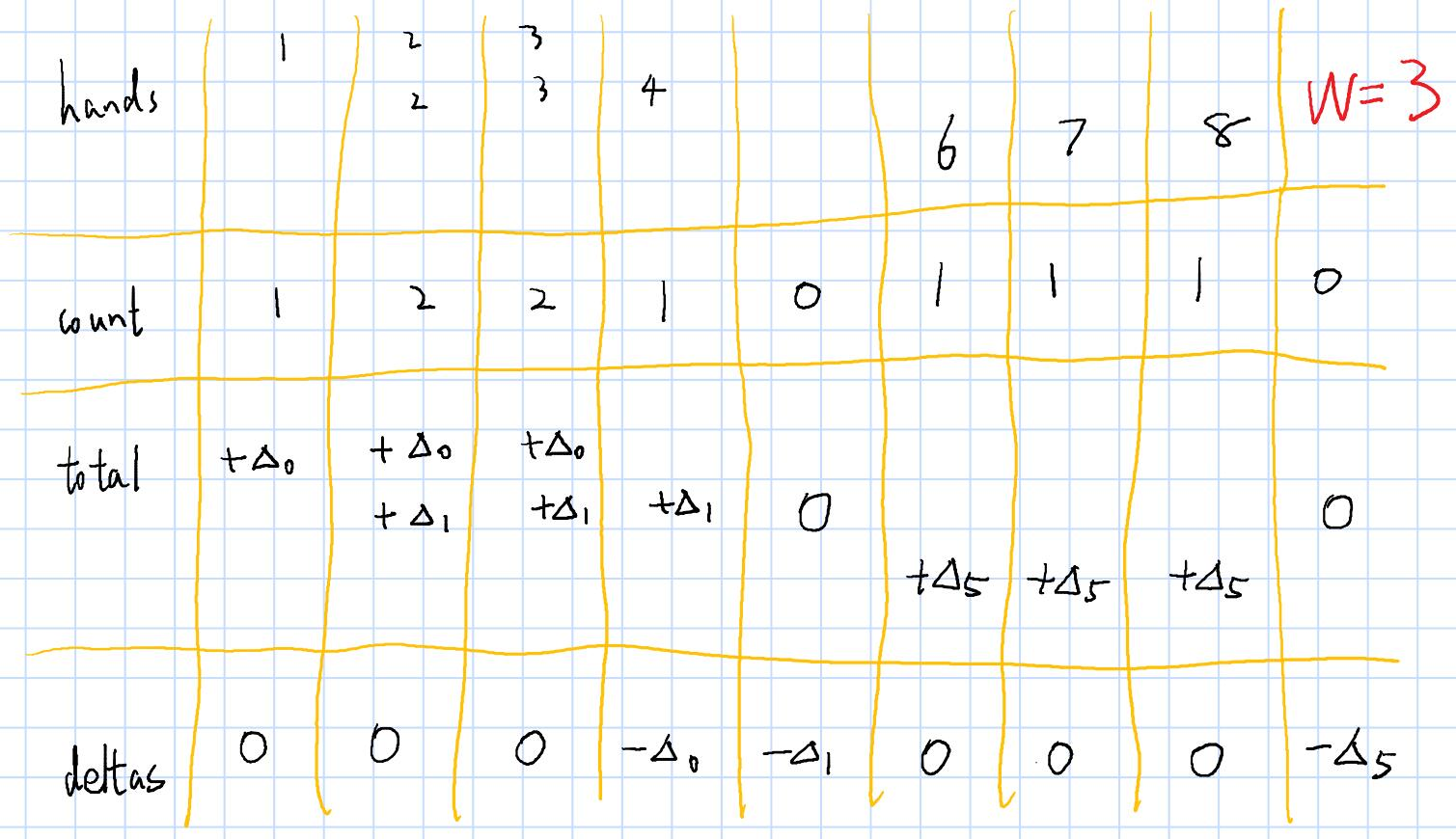【每日算法Day 103】老题新做，几乎不会有人想到的解法，它来了

## 题目链接

LeetCode 846. 一手顺子

## 题目描述

• 1 <= hand.length <= 10000
• 0 <= hand[i] <= 10^9
• 1 <= W <= hand.length

输入：
hand = [1,2,3,6,2,3,4,7,8], W = 3

true

输入：
hand = [1,2,3,4,5], W = 4

false

## 题解• 然后从小到大遍历每一张牌，首先 1 只有一张，那么如果它和后面牌能构成顺子，那么 2, 3 至少要有一张才行，于是 total 数组后面两个位置都加上 1
• 然后遍历到 2 ，因为 2 的数量是大于该位置处的 total 值的，所以 2 的数量足够满足前面的牌顺子要求。此外 2 还会多出一张，那么后面两个位置至少要有一张牌才行，于是 total 后面两个位置再加上 1
• 然后遍历 3, 4 ，发现数量正好都等于 total ，那说明它俩正好和前面的牌构成顺子，一点都不会多余。
• 然后遍历到 0 了，这就说明和前面的牌断开了。如果这时候 total 不为 0 ，就说明中间缺失了一些牌，前面存在顺子没法补足结尾。而如果最开始没有填充 0 的话，就没有办法判断这里的牌是否和前面连续的，你就有可能把 6 这张牌直接接到 4 后面组成顺子了。
• 然后遍历 6, 7, 8 同理，在对应位置处更新 total 就行了。
• 最后遍历 0 ，发现 total 也是 0 ，那就说明整副牌可以构成顺子，完美！

• 首先遍历 1 ，因为 1 只有一张，那么如果它和后面牌能构成顺子，那么 2, 3 至少要有一张才行。但是这里我们不对这几张牌的 total 加上一，而是在这个顺子结尾的下一张牌处的 deltas 减去 1
• 然后遍历 2 ，那么这时候没有 total 了，怎么计算应该扣除多少前面顺子需要的 2 呢？其实只需要用前一张牌的牌数加上当前的 deltas 值就行了。为什么呢？前面一张牌有多少张，你当前这张就得至少有那么多去构成顺子，但是如果前面一张牌是某些顺子的结尾，你还得扣掉一些，而扣掉的数值正好就是当前的 deltas ，这在前面顺子的开头处已经记录过了。
• 后面操作类似，就不详细阐述了。

## 代码

### 暴力更新（c++）

class Solution {
public:
bool valid(vector<int> &count, int W) {
int n = count.size();
vector<int> total(n, 0);
for (int i = 0; i < n; ++i) {
if (count[i] > total[i]) {
int delta = count[i] - total[i];
for (int j = i; j < i+W && j < n; ++j) total[j] += delta;
} else if (count[i] < total[i]) {
return false;
}
}
return true;
}

bool isNStraightHand(vector<int>& hand, int W) {
int n = hand.size();
if (W == 1) return true;
if (n%W) return false;
sort(hand.begin(), hand.end());
vector<int> count;
int i = 0, j = 0;
while (i < n) {
while (j < n && hand[i] == hand[j]) j++;
count.push_back(j-i);
if (j >= n) break;
else if (hand[j] != hand[j-1]+1) count.push_back(0);
i = j;
}
count.push_back(0);
return valid(count, W);
}
};

### 优化（c++）

class Solution {
public:
bool valid(vector<int> &count, int W) {
int n = count.size(), pre = 0;
vector<int> deltas(n, 0);
for (int i = 0; i < n; ++i) {
pre += deltas[i];
if (pre < count[i]) {
int delta = count[i] - pre;
pre = count[i];
if (i + W < n) deltas[i+W] -= delta;
} else if (pre > count[i]) {
return false;
}
}
return true;
}

bool isNStraightHand(vector<int>& hand, int W) {
int n = hand.size();
if (W == 1) return true;
if (n%W) return false;
sort(hand.begin(), hand.end());
vector<int> count;
int i = 0, j = 0;
while (i < n) {
while (j < n && hand[i] == hand[j]) j++;
count.push_back(j-i);
if (j >= n) break;
else if (hand[j] != hand[j-1]+1) count.push_back(0);
i = j;
}
count.push_back(0);
return valid(count, W);
}
};转载规则

《【每日算法Day 103】老题新做，几乎不会有人想到的解法，它来了》 韦阳 采用 知识共享署名 4.0 国际许可协议 进行许可。
上一篇【每日算法Day 104】偷电瓶的周某今天放出来了，还不赶紧做这道题防范一下！

2020-04-18【每日算法Day 102】美团 AI 平台算法工程师面试编程题

2020-04-16
目录